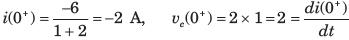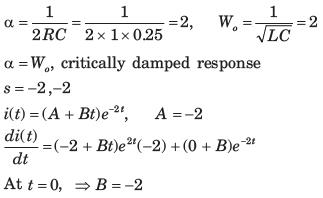Courses

# Test: Transient Response Of Dc And Ac Networks Part - 2

## 10 Questions MCQ Test Networking Theory | Test: Transient Response Of Dc And Ac Networks Part - 2

Description
This mock test of Test: Transient Response Of Dc And Ac Networks Part - 2 for Electrical Engineering (EE) helps you for every Electrical Engineering (EE) entrance exam. This contains 10 Multiple Choice Questions for Electrical Engineering (EE) Test: Transient Response Of Dc And Ac Networks Part - 2 (mcq) to study with solutions a complete question bank. The solved questions answers in this Test: Transient Response Of Dc And Ac Networks Part - 2 quiz give you a good mix of easy questions and tough questions. Electrical Engineering (EE) students definitely take this Test: Transient Response Of Dc And Ac Networks Part - 2 exercise for a better result in the exam. You can find other Test: Transient Response Of Dc And Ac Networks Part - 2 extra questions, long questions & short questions for Electrical Engineering (EE) on EduRev as well by searching above.
QUESTION: 1

### In the circuit of fig. P1.6.23 the switch is opened at t = 0 after long time. The current iL (t) for t > 0 is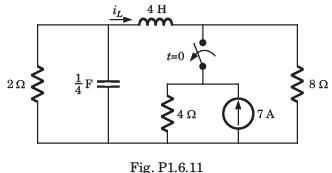Solution: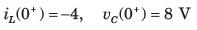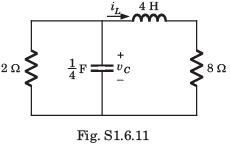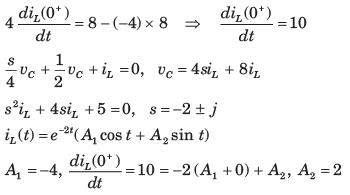QUESTION: 2

### In the circuit shown in fig. P1.6.12–14 all initial condition are zero.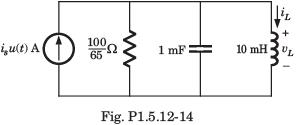Q. If is (t) = 1 A, then the inductor current iL (t) is

Solution: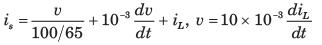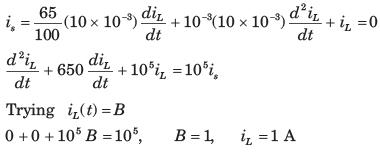QUESTION: 3

### In the circuit shown in fig. P1.6.12–14 all initial condition are zero.Q. If is (t) = 0.5 A, then iL (t) is

Solution:
QUESTION: 4

In the circuit of fig. P1.6.24 switch is moved from position a to b at t = 0. The iL(t) for t > 0 is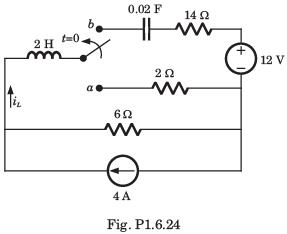Solution: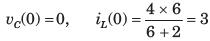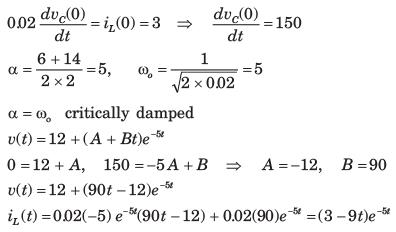QUESTION: 5

In the circuit shown in fig. P1.6.25 a steady state has been established before switch closed. The i(t) for t > 0 is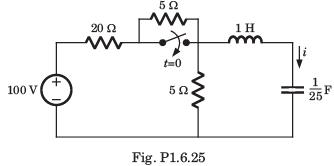Solution: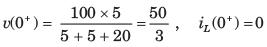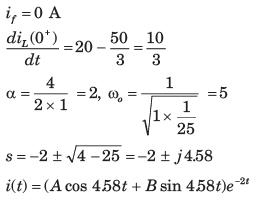QUESTION: 6

The switch is closed after long time in the circuit of fig. P1.6.26. The v(t) for t > 0 is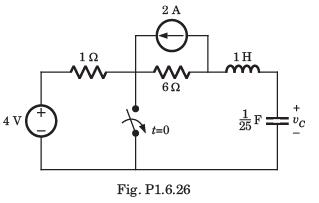Solution: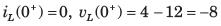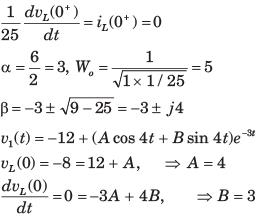QUESTION: 7

i(t) = ?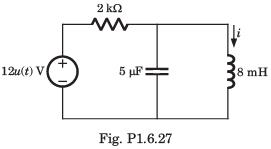Solution: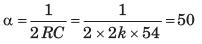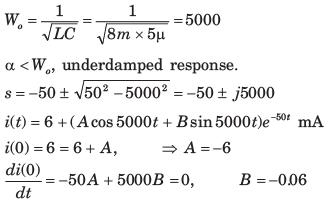QUESTION: 8

In the circuit of fig. P1.6.28 i(0) = A and v(0) =0.The current i(t) for t > 0 is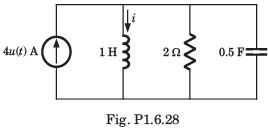Solution: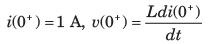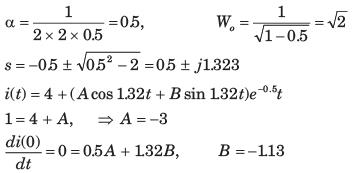QUESTION: 9

In the circuit of fig. P1.6.29 a steady state has been established before switch closed. The vo(t) for t > 0 is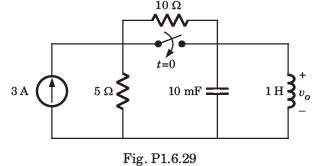Solution: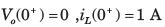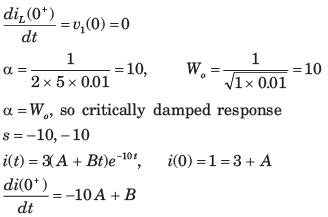QUESTION: 10

In the circuit of fig. P1.6.30 a steady state has been established before switch closed. The i(t) for t > 0 is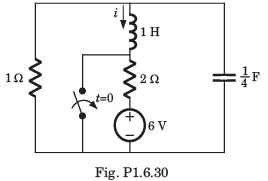Solution: